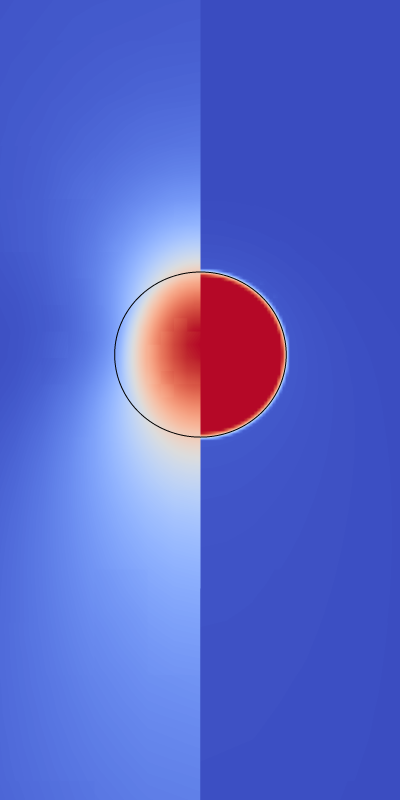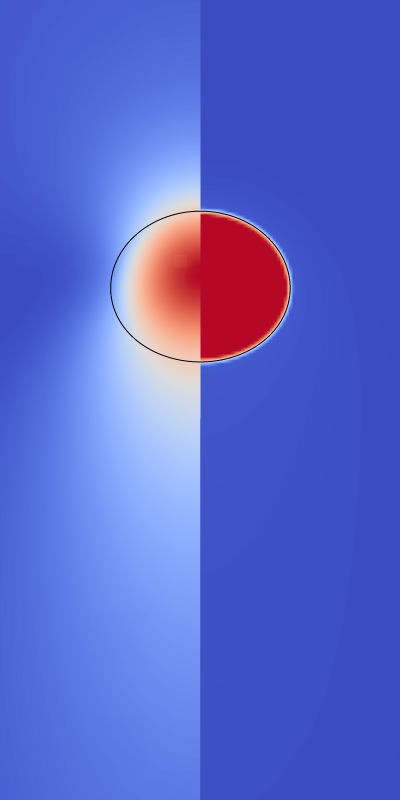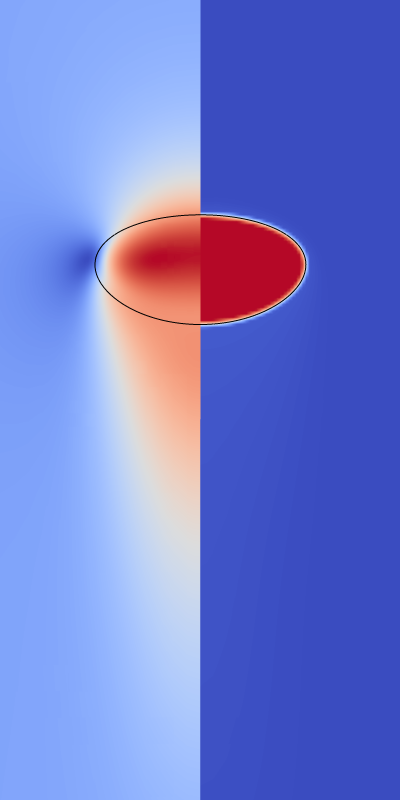# Soluble gas diffusing from a rising bubble

This is the example discussed in section 3.3.2 of Farsoiya et al., 2021.

The four cases illustrated below are considered. The left-half of each figure is the vertical velocity component and the right-half the tracer concentration when close to the stationary regime.Case 1 Case 2 Case 3 Case 4

The Sherwood number characterises the gas diffusion from the bubble to the liquid. The computed Sherwood number is compared to the theory of Levich, 1962.

alpha_c = 1.0/30
r0 = 1.

sherwood(D) = (ci = $2/$5,  \
co = $3/$6, \
area = $7, \ dtr = (tr -$2)/0.01, \
tr = $2, \ Sh = dtr/area/(ci*alpha_c - co)*2.*r0/D) # Levich formula to calculate transfer rate using terminal velocity levich(ut, D) = 2.*sqrt(D*ut/2./r0/pi)*2.*r0/D # get the rise velocity averaged for the last steps array ut array et = [ 140, 250, 250, 150 ] do for [i in "1 2 3 4"] { stats 'log' index 'case '.i u 1:4 every ::et nooutput ut[i] = STATS_mean_y } array D = [ 0.4472, 0.5029, 0.17416, 0.08944 ] tr = 0. set key center right set xlabel 't U/d_0' set ylabel 'Sh' set xrange [0.01:4] set yrange [0:14] plot for [i = 1:4] \ 'log' index 'case '.i u ($1*ut[i]/2./r0):(sherwood(D[i])) \
w l t 'Axi Case '.i lt i,				       \
for [i = 1:4] levich(ut[i], D[i]) w l t '' lt i dt 2Sherwood number vs time (script)

 [farsoiya2021] Palas Kumar Farsoiya, Stéphane Popinet, and Luc Deike. Bubble-mediated transfer of dilute gas in turbulence. Journal of Fluid Mechanics, 920(A34), June 2021. [ DOI | http | .pdf ] [levich1962] Veniamin Grigorʹevich Levich. Physicochemical hydrodynamics. Prentice-Hall Inc., 1962.
#include "axi.h"
#include "navier-stokes/centered.h"
#include "two-phase.h"
#include "navier-stokes/conserving.h"
#include "tension.h"
#include "reduced.h"
#include "henry.h"
#include "view.h"

scalar c[], * stracers = {c};
double box_size = 20.;
double conc_liq1 = 0, conc_gas1 = 1.;
double end_time = {7.5,3,3,2};

int MAXLEVEL, dcase = 1;

int main (int argc, char **argv)
{
size (box_size);

MAXLEVEL = 9;
#if !TREE
N = 1 << MAXLEVEL;
#endif

rho1 = 1.;
rho2 = 0.01;
c.alpha = 1./30.;
TOLERANCE = 1e-4 [*];

for (dcase = 1; dcase <= 4; dcase++) {
switch (dcase) {
case 1:
c.D1 = 0.4472;
f.sigma = 10.0;
G.x = - 2.5;
break;
case 2:
c.D1 = 0.5029;
f.sigma = 10.0;
G.x = - 7.8125;
break;
case 3:
c.D1 = 0.17416;
f.sigma = 1.0;
G.x = - 10.0;
break;
case 4:
c.D1 = 0.08944;
f.sigma = 10.0;
G.x = - 7.8125;
break;
default:
fprintf (stderr, "Error: must specify case\n");
exit (1);
}

mu1 = c.D1;
mu2 = c.D1/20.;
c.D2 = c.D1*100.;

fprintf (stderr, "\n\n# case %d\n", dcase);
run();
}
}

event init (t = 0)
{
#if TREE
refine (sq(2.*bubble_radius) - sq(x - box_size*0.2) - sq(y) > 0 &&
level < MAXLEVEL);
#endif

fraction (f, - (sq(bubble_radius) - sq(x - box_size*0.2) - sq(y)));

foreach()
c[] = conc_liq1*f[] + conc_gas1*(1. - f[]);
}

#if TREE
maxlevel = MAXLEVEL);
}
#endif

event extract (t = 0; t += 0.01; t <= end_time[dcase-1])
{
double yb = 0., vb = 0., vbx = 0., area = 0., ci = 0., co = 0.;
foreach (reduction(+:yb) reduction(+:vb) reduction(+:vbx)
reduction(+:ci) reduction(+:co)
reduction(+:area)) {
double dvb = (1. - f[])*dv();
vb += dvb;          // volume of the bubble
yb += x*dvb;	// location of the bubble
vbx += u.x[]*dvb;	// bubble velocity

ci += c[]*(1. - f[])/(f[]*c.alpha + (1. - f[]))*dv();
co += c[]*f[]*c.alpha/(f[]*c.alpha + (1. - f[]))*dv();

if (f[] > 1e-6 && f[] < 1. - 1e-6) {
coord n = interface_normal (point, f), p;
double alpha = plane_alpha (f[], n);
// area of the bubble interface
area += y*pow(Delta, dimension - 1)*plane_area_center (n, alpha, &p);
}
}

if (i == 0)
fprintf (stderr, "t ci co vbx vb vbo area dt\n");
fprintf (stderr,"%g %g %g %g %g %g %g %g\n",
t,
ci*2.*pi, co*2.*pi,
vbx/vb, 2.*pi*vb, 2.*pi*statsf(f).sum, 2.*pi*area,
dt);
}

event pictures (t = end)
{
char name;
#if 0
sprintf (name, "dump-%d", dcase);
dump (name);
#endif

double ty[] = { - 0.6, - 0.5, - 0.5, - 0.5};
view (fov = 9, quat = {0.707,0.707,0,0},
ty = ty[dcase - 1],
width = 400, height = 800);
squares ("c", spread = -1, linear = true, map = cool_warm);
draw_vof ("f");
mirror ({0,1}) {
squares ("u.x", spread = -1, linear = true, map = cool_warm);
draw_vof ("f");
}
sprintf (name, "final-%d.png", dcase);
save (name);
}